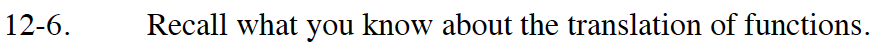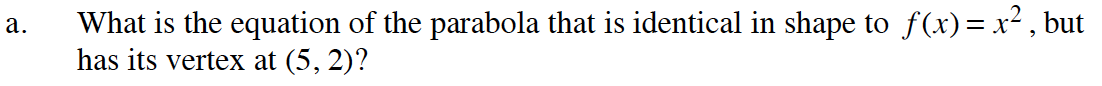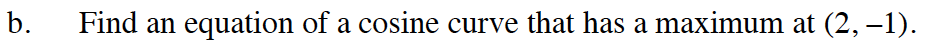### Home > CALC > Chapter 12 > Lesson 12.1.1 > Problem12-6

12-6.
1. Recall what you know about the translation of functions. Homework Help ✎

1. What is the equation of the parabola that is identical in shape to f(x) = x2 , but has its vertex at (5, 2)?

2. Find an equation of a cosine curve that has a maximum at (2, −1).Recall that vertex form for a parabola is y = a(xh)2 + k.The function f(x) = cos(x) has a maximum point at (0, 1).
How far does the original function need to be translated horizontally? Vertically?